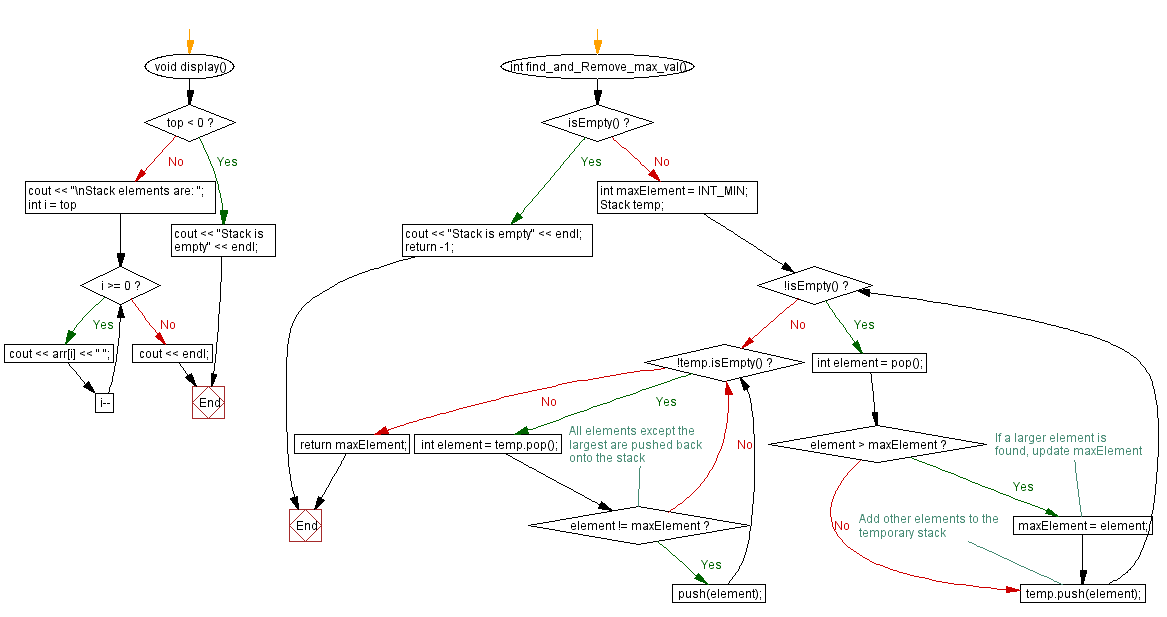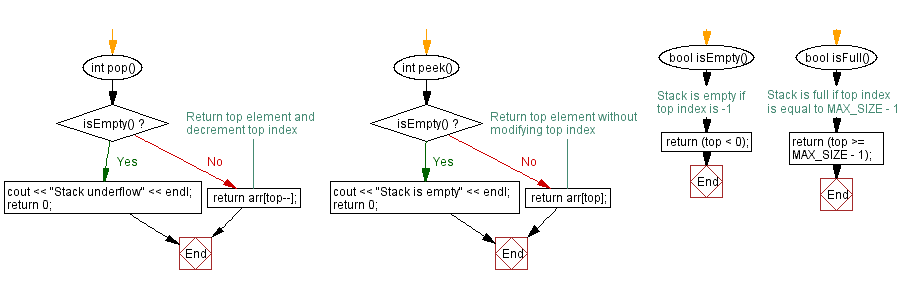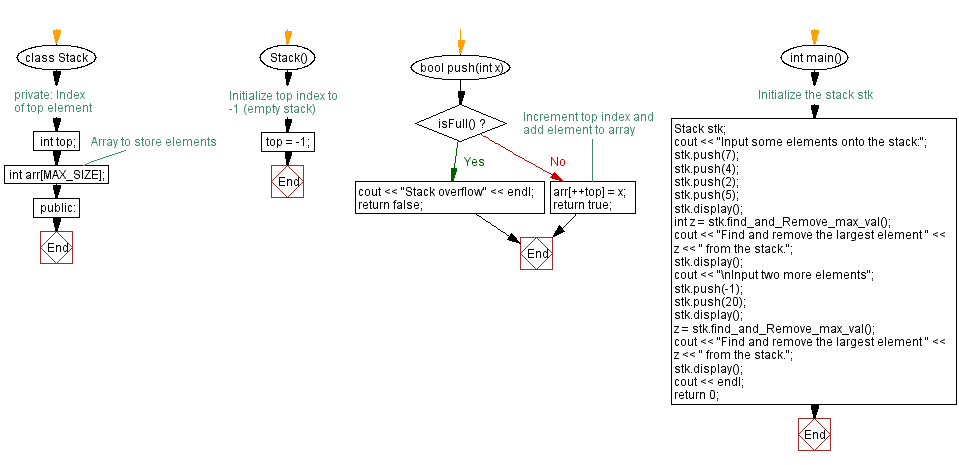﻿ C++ Find and remove the largest element in a stack

# C++ Stack Exercises: Find and remove the largest element in a stack

## C++ Stack: Exercise-10 with Solution

Write a C++ program to find and remove the largest element in a stack.

Test Data:
Input some elements onto the stack:
Stack elements are: 5 2 4 7
Find and remove the largest element 7 from the stack.
Stack elements are: 5 2 4

Sample Solution:

C++ Code:

``````#include <iostream>

using namespace std;
#define MAX_SIZE 15 // Maximum size of stack

class Stack {
private:
int top; // Index of top element
int arr[MAX_SIZE]; // Array to store elements
public:
Stack() {
top = -1; // Initialize top index to -1 (empty stack)
}

bool push(int x) {
if (isFull()) {
cout << "Stack overflow" << endl;
return false;
}
// Increment top index and add element to array
arr[++top] = x;
return true;
}

int pop() {
if (isEmpty()) {
cout << "Stack underflow" << endl;
return 0;
}
return arr[top--];
}

int peek() {
if (isEmpty()) {
cout << "Stack is empty" << endl;
return 0;
}
return arr[top];
}

bool isEmpty() {
// Stack is empty if top index is -1
return (top < 0);
}

bool isFull() {
// Stack is full if top index is equal to MAX_SIZE - 1
return (top >= MAX_SIZE - 1);
}

void display() {
if (top < 0) {
cout << "Stack is empty" << endl;
return;
}
cout << "\nStack elements are: ";
for (int i = top; i >= 0; i--)
cout << arr[i] << " ";
cout << endl;
}

int find_and_Remove_max_val() {
if (isEmpty()) {
cout << "Stack is empty" << endl;
return -1;
}

int maxElement = INT_MIN;
Stack temp;
while (!isEmpty()) {
int element = pop();
if (element > maxElement) {
//If a larger element is found, update maxElement
maxElement = element;
}
// Add other elements to the temporary stack
temp.push(element);
}

while (!temp.isEmpty()) {
int element = temp.pop();
// All elements except the largest are pushed back onto the stack
if (element != maxElement) {
push(element);
}
}
return maxElement;
}

};

int main() {
//Initialize the stack stk
Stack stk;
cout << "Input some elements onto the stack:";
stk.push(7);
stk.push(4);
stk.push(2);
stk.push(5);
stk.display();
int z = stk.find_and_Remove_max_val();
cout << "Find and remove the largest element " << z << " from the stack.";
stk.display();
cout << "\nInput two more elements";
stk.push(-1);
stk.push(20);
stk.display();
z = stk.find_and_Remove_max_val();
cout << "Find and remove the largest element " << z << " from the stack.";
stk.display();
cout << endl;
return 0;
}
``````

Sample Output:

```Input some elements onto the stack:
Stack elements are: 5 2 4 7
Find and remove the largest element 7 from the stack.
Stack elements are: 5 2 4

Input two more elements
Stack elements are: 20 -1 5 2 4
Find and remove the largest element 20 from the stack.
Stack elements are: -1 5 2 4
```

Flowchart:CPP Code Editor: